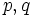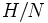# Group satisfying Oliver's condition

A group satisfying Oliver's condition is a finite group$G$ satisfying the following equivalent conditions:
•$G$ is a$q$-group extension of a cyclic extension of a$p$-group: There exist primes$p,q$ (possibly equal) and subgroups$N \le H \le G$, such that the following hold.$N$ is a normal subgroup of$H$,$H$ is a normal subgroup of$G$,$G/H$ is a$q$-group,$H/N$ is a cyclic group, and$N$ is a$p$-group. By a$p$-group (resp.,$q$-group), we mean a group of prime power order where the underlying prime is$p$ (resp.,$q$).
• Any action of$G$ on a contractible finite simplicial complex has a fixed face (in the geometric realization, a fixed point).